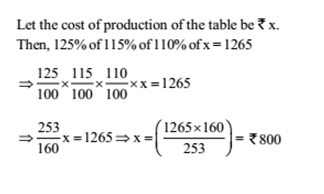# RRB ALP 2018 Practice Test Papers | Arithmetic Questions (Day-33)

Dear Aspirants, Here we have given the Important RRB ALP & Technicians Exam 2018 Practice Test Papers. Candidates those who are preparing for RRB ALP 2018 can practice these Arithmetic Questions to get more confidence to Crack RRB 2018 Examination.

[WpProQuiz 2042]

Click “Start Quiz” to attend these Questions and view Explanation

1. The sum of two numbers is 28 /25 of the first number. The second number is what percent of the first?

(a) 12%

(b) 14%

(c) 16%

(d) 18%

1. A ship is moving at a speed of 30 km/hr. To know the depth of the ocean beneath it, it sends a radiowave which travels at a speed 200 m/s. The ship receives the signal after it has moved 500 m. The depth of the ocean is

(a) 6 km

(b) 12 km

(c) 6 m

(d) 8 km

1. A wooden box of dimension 8 metre × 7 metre × 6 metre is to carry rectangular boxes of dimensions 8 cm × 7 cm × 6 cm. The maximum number of boxes that can be carried in 1 wooden box is

(a) 7500000

(b) 9800000

(c) 1200000

(d) 1000000

1. If the cost price is 96% of the selling price, then what is the profit percent?

(a) 4.5%

(b) 4.2%

(c) 4%

(d) 3.8%

1. A cyclist covers a distance of 750 m in 2 min 30 sec. What is the speed in km/h of the cyclist?

(a) 18 km/h

(b) 15 km/h

(c) 20 km/h

(d) None of these

1. Four runners started running simultaneously from a point on a circular track. They took 200 seconds, 300 seconds, 360 seconds and 450 seconds to complete one round. After how much time they meet at the starting point for the first time?

(a) 1800 seconds

(b) 3600 seconds

(c) 2400 seconds

(d) 4800 seconds

1. What is the smallest value that must be added to 709, so that the resultant is a perfect square?

(a) 8

(b) 12

(c) 20

(d) 32

1. A batsman in his 12th innings makes a score of 65 and thereby increases his average by 2 runs. What is his average after the 12th innings if he had never been ‘not out’?

(a) 42

(b) 43

(c) 44

(d) 45

1. If 10% of an electricity bill is deducted, Rs. 45 is still to be paid. How much was the bill?

(a) Rs. 50

(b) Rs. 60

(c) Rs. 55

(d) None of these

1. If the manufacturer gains 10%, the wholesale dealer 15% and the retailer 25%, then find the cost of production of a table, the retail price of which is Rs. 1265?

(a) Rs. 800

(b) Rs. 1000

(c) Rs. 900

(d) Rs. 600

Let the numbers be x and y. Then,

x+y=28/25 x

=>y= 3/25 x

y/x=3/25×100=12%

Speed = 30 km/h = 30 x5/18= 25/3 m/ s

1 Distance covered by ship = 500m

Time = 500X3/25= 60sec

Distance covered by wave = 60 × 200 = 12000 m

Depth of sea = 12000/1000= 12km

Maximum number of boxes =  800x 700 x600/( 8 x7x 6)  = 1000000

Let S.P. = Rs. 100. Then, C.P. = Rs. 96; Profit = Rs. 4.

Profit= 4/96×100=4.17%

Speed = 750/150 m/ sec =5 m/ sec

=5×18/5=18 km/hr

Required time = LCM of 200, 300, 360 and 450 seconds = 1800 seconds.

According to question 26 <√ 709<  27

Now, (27)2 = 729

729 – 709 = 20

20 must be added to 709 to make it a perfect square.

Let ‘x’ be the average score after 12th innings

12 x = 11 × (x – 2) + 65

x = 43

Let the bill be Rs. x. Then 90% of x = 45 => 45x 100/90 = 50RRB ALP 2018 Practice Test Papers | Arithmetic Questions (Day-1)

RRB ALP 2018 Practice Test Papers | Arithmetic Questions (Day-2)

RRB ALP 2018 Practice Test Papers | Arithmetic Questions (Day-3)

RRB ALP 2018 Practice Test Papers | Arithmetic Questions (Day-4)

RRB ALP 2018 Practice Test Papers | Arithmetic Questions (Day-5)

RRB ALP 2018 Practice Test Papers | Arithmetic Questions (Day-6)

RRB ALP 2018 Practice Test Papers | Arithmetic Questions (Day-7)

RRB ALP 2018 Practice Test Papers | Arithmetic Questions (Day-8)

RRB ALP 2018 Practice Test Papers | Arithmetic Questions (Day-9)

RRB ALP 2018 Practice Test Papers | Arithmetic Questions (Day-10)

RRB ALP 2018 Practice Test Papers | Arithmetic Questions (Day-11)

RRB ALP 2018 Practice Test Papers | Arithmetic Questions (Day-12)

RRB ALP 2018 Practice Test Papers | Arithmetic Questions (Day-13)

RRB ALP 2018 Practice Test Papers | Arithmetic Questions (Day-14)

RRB ALP 2018 Practice Test Papers | Arithmetic Questions (Day-15)

RRB ALP 2018 Practice Test Papers | Arithmetic Questions (Day-16)

RRB ALP 2018 Practice Test Papers | Arithmetic Questions (Day-17)

RRB ALP 2018 Practice Test Papers | Arithmetic Questions (Day-18)

RRB ALP 2018 Practice Test Papers | Arithmetic Questions (Day-19)

RRB ALP 2018 Practice Test Papers | Arithmetic Questions (Day-20)

RRB ALP 2018 Practice Test Papers | Arithmetic Questions (Day-21)

RRB ALP 2018 Practice Test Papers | Arithmetic Questions (Day-22)

RRB ALP 2018 Practice Test Papers | Arithmetic Questions (Day-23)

RRB ALP 2018 Practice Test Papers | Arithmetic Questions (Day-24)

RRB ALP 2018 Practice Test Papers | Arithmetic Questions (Day-25)

RRB ALP 2018 Practice Test Papers | Arithmetic Questions (Day-26)

RRB ALP 2018 Practice Test Papers | Arithmetic Questions (Day-27)

RRB ALP 2018 Practice Test Papers | Arithmetic Questions (Day-28)

RRB ALP 2018 Practice Test Papers | Arithmetic Questions (Day-29)

RRB ALP 2018 Practice Test Papers | Arithmetic Questions (Day-30)

RRB ALP 2018 Practice Test Papers | Arithmetic Questions (Day-31)

RRB ALP 2018 Practice Test Papers | Arithmetic Questions (Day-32)